Relation between the Gravitational and Magnetic Fields | OMICS International
Journal of Physical Mathematics

# Relation between the Gravitational and Magnetic Fields

Baixauli JG*

Independent Researcher, Spain

*Corresponding Author:
]Baixauli JG
Independent Researcher
Spain
Tel: 023955775555
E-mail: [email protected]

Received Date: March 07, 2016; Accepted Date: April 26, 2016; Published Date: May 03, 2016

Citation: Baixauli JG (2016) Relation between the Gravitational and Magnetic Fields. J Phys Math 7: 169. doi: 10.4172/2090-0902.1000169

Copyright: © 2016 Baixauli JG. This is an open-access article distributed under the terms of the Creative Commons Attribution License, which permits unrestricted use, distribution, and reproduction in any medium, provided the original author and source are credited.

Visit for more related articles at Journal of Physical Mathematics

#### Abstract

Quantum and relativistic phenomena can be explained by the hypothesis that both the universe and the elementary particles are formed by atoms’ four spatial dimensions. It is also possible to deduce and calculate properties of elementary particles, such as mass, electric charge, spin, radio, etc. Based on this hypothesis, the fundamental constants can be calculated depending only on the speed of light c. Moreover, the Schrodinger equation can be deduced and the equality of inertial and gravitational mass can be demonstrated. The aim of this article is to establish a relationship between electron mass and electron charge, and thereby calculate the electrical charge depending on the mass of the electron, Planck's constant h and the speed of light c. Through this relationship, extended to the gravitational and magnetic fields, it is possible to establish a relationship between the constants of both fields.

#### Keywords

Gravitational and magnetic fields; Quantum gravity

#### Introduction

Magnetic and electrical phenomena were already known in Ancient Greece. In the 17th and 18th Centuries, electromagnetic phenomena were studied separately. James Clerk Maxwell described the electric and magnetic fields using a set of equations in 1861, unifying the two fields into one: the electromagnetic field. In Newtonian physics, the gravitational field is defined as the force per unit mass that experiences a point particle in the presence of a mass. In general relativity, gravity is due to the curvature of space time. The presence of a mass curves space time, causing bodies to move along those lines curves denominated geodesic. General relativity assumes that space time is continuous. However, there is no experimental evidence for it. Are space and time continuous? Or are we only convinced of that continuity as a result of education? In recent years, both physicists and mathematicians have asked whether it is possible that space and time are discrete. If we could probe to size scales that were small enough, would we see “atoms” of space, irreducible pieces of volume that cannot be broken into anything smaller? . Quantification of space time allows us to distinguish elementary particles from each other in a simple and natural way [2,3].

Minimum volume, length or area are measured in the units of Planck . Theories related to quantum gravity, such as string theory and doubly special relativity, as well as black hole physics, predict the existence of a minimum length [4,5]. The familiar concept of a spacetime continuum implies that it should be possible to measure always smaller and smaller distances without any finite limit . Heisenberg, who insisted on expressing quantum mechanical laws in terms of measurable observables, questioned already the validity of this postulate . Heisenberg said that physics must have a fundamental length scale, and with Planck's constant h and the speed of light c, allow the derivation of the masses of the particles [7,8]. Planck’s length has been considered as the shortest distance having any physical meaning. A fundamental (minimal) length scale naturally emerges in any quantum theory in the presence of gravitational effects that accounts for a limited resolution of space-time. As there is only one natural length scale we can obtain by combining gravity (G), quantum mechanics (â��) and special relativity (c), this minimal length is expected to appear at the Planck scale . Padmanabhan shows that the Planck length provides a lower limit of length in any suitable physical space-time [10,11]. It is impossible to construct an apparatus which will measure length scales smaller than Planck length. These effects exist even in flat space-time because of vacuum fluctuations of gravity . Quantum particles in discrete spacetime are studied in relation to relativistic dynamics [12,13]. Farrelly and Short studied the causal evolution of a single particle in discrete spacetime .

There is evidence of discrete structures on the largest scales, for example superclusters and the redshift . Cowan already said in 1969 that redshift can only occur with discrete values . This was subsequently confirmed by Karlsson .

Relations between Rest Mass and Electric Charge

The hypothesis is that the universe is composed of four spatial dimensions Planck atoms, with two possible states: rest state and rotational movement. Rest atoms are empty space, and the rotational motion of the atoms gives rise to different properties of the particles. Of the four dimensions, three are seen as space (x, y and z) and the fourth (u=ct) is observed as time. The 4D Planck atoms are atoms of space and time that Smolin says. To simplify the drawing (Figure 1), only three dimensions are considered: r(x,y) and u. If space is made up of Planck-size atoms, each Planck atom can only be resting or turning on itself. The rotations can be in three-dimensional space or in the fourth dimension. The rotational energy in the fourth dimension gives rise to the rest mass and period originating the electric charge.

The energy of the particle can be expressed as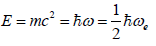(1)

Where m is the rest mass of the particle, c the speed of light, Ä§ the reduced Planck constant (h/2π) and ωu rotation in the fourth dimension (Figure 2). A 4D Planck atom may rotate both in three dimensional space and in the fourth dimension (u, Figure 2), which results in the following combinations:

• Zero rotations. The vacuum space.

• One spatial rotation ωe. Neutrinos.

• One rotation in the fourth dimension ωu Photons.

• Two rotations, one spatial rotation (ωe) and one rotation in the fourth dimension ωu. Electrons and quarks of the first generation.

Equation (1) can be expressed in terms of period (wu=2 π/Tu), resulting in: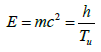(2)

The electric charge will be due to the rotation (one of three possible rotations) or therefore, the electric charge can be expressed as: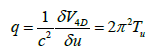(3)

Substituting (Tu) in Equation (2), the following is obtained: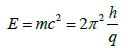(4)

Where m is the electron rest mass, and electric charge is in seconds.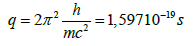(5)

To preserve the units, it is only necessary to multiply Equation (2) by the current unit (I=1 A). A coulomb is an arbitrary unit of electrical charge. Current theory allows one to measure the electrical charge but not to explain it. Simply, the electric charge is defined as an intrinsic or fundamental property of matter.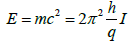(6)

The rest mass and charge of the electron were calculated above, according to Planck's constant h and the speed of light c .

Corrections

When measurements are made, three factors should be taken into account to avoid errors in the value of the parameter measured in the laboratory or mathematically calculated.

These factors are:

Light refraction index (n) some parameters are measured in a vacuum and others in the laboratory. Everything depends on the speed of light, so it will be necessary to take into account the light refraction index in the atmosphere.

Special Relativity (SR) Assuming that the Earth moves to 21000 km/s, due to the movement of the Sun around the galaxy, the movement of the galaxy in the direction of the Virgo cluster and other possible movements due to the gravitational attraction, should be taken into account.

Gravitational system General relativity establishes that time elapses more slowly in a gravitational system than far from the gravitational potential.

This difference over time relative to gravitational potential is: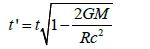(7)

Where t is the time measured away from the gravitational system. Taking into account n and SR, and I0 current of 1 A becomes: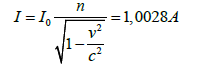(8)

Measures should note the time and the speed of light in the laboratory, because the intensity is defined as coulombs per unit time (I=q/t). Then Equation (5) becomes: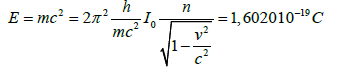(9)

Where h=6.6260700410-34 Js, m=9.109383560 10-31 kg, c=299792458 m s-1, n=1.00029260 and I0=1 A.

Although both electromagnetic field and the gravitational field are well known, it may be interesting to review some basic concepts of both fields. Specifically, referring to the orbits.

Electromagnetic Field

In classical physics the electric field is produced by the accumulation of electrical charge, while the magnetic field is due to electric current. In relativistic physics, an observer at rest with respect to a reference system will measure a different value than that an observer moving relative to the reference system mentioned above would measure.It has been shown that the electric charge is the time it takes the Planck atom to spin in the fourth dimension, and that the mass is the energy of this rotation or the magnetic field energy. Therefore, the electromagnetic and gravitational fields must be related, as are the mass and electric charge.

Magnetic Orbits

When a negative charge q moving with velocity v penetrates a magnetic field B, the charge is subject to a force. If the field is perpendicular to the velocity, the modulus of the magnetic force can be calculated with Equation (10) (Figure 3):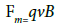(10)

The radius of the circular orbit is determined by the condition that the centripetal force of magnetic origin is compensated by the centrifugal force, then: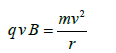(11)

#### Gravitational Orbits

If a body of mass m, moving with velocity v, penetrates a gravitational field g, it will be subject to an attractive force. If the kinetic and potential energies remain constant over time, the body will describe a circular orbit (Figure 4).

The gravitational force will go in the direction of the field and the modulus will be: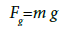(12)

To calculate the radius of the orbit, the dynamics of uniform circular motion is applied (i.e. Newton’s Second Law):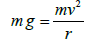(13)

Relationship between Fields

The units of the gravitational and magnetic field are m.s-2 and kg·s-2.A-1 respectively. If both fields produce the same phenomenon, why do they not have the same units? We raise the issue conversely: suppose that a particle of mass m and charge q+ describes a circular orbit with speed v, about X field; find the term and nature of the field X. Considering the mass-dependent phenomenon, it is a gravitational field, but if we consider that the attraction depends on the electric charge, a magnetic field is obtained. From Equations (11) and (13):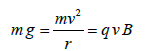(14)

And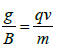(15)

If g and B are equal to 1 (g=B=1):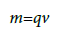(16)

In a universe formed by four spatial dimensions (Planck spheres) that expands at the speed of light, it is clear that the previous Equation (16) must hold, because there are only space and movement. The movement can be observed in different ways, such as time, frequency, electric charge, rest mass, etc. If the electric charge is the time that a Planck atom takes to turn one spin in the fourth dimension, the rest mass must be related to the conditions of Planck.

#### Conditions of Planck

If Equation (15) is applied under the conditions of Planck, it is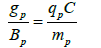(17)

Where qp is the Planck charge and mp the Planck mass. Gravitational and Coulomb forces are equal under Planck conditions, then: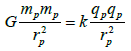(18)

Where k is Coulomb constant and rp is the Planck radius, which is the Planck atom diameter. Then: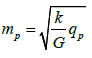(19)

Multiplying and dividing by the speed of light: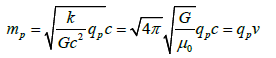(20)

As the rotation of the Planck atom decreases, the speed decreases until the minimum energy state. This state matches the conditions of the electron in the free state, and its speed: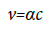(21)

Considering both Equations (23) and (24):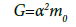(22)

6.67408·10-11=(7.2973525664 10-3)2 x 1.2566370614·10-6 =6.69176·10-11

Obviously, in this equation the units do not match, because neither quantum mechanics nor general relativity explain rest mass or electric charge. These theories only assign arbitrary units to them and consider them independent intrinsic properties.

If it is intended to recover the typical units of these parameters, Equation (22) can be simply multiplied as follows: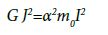(23)

Where J is the linear mass density (J=1 kg m-1) and current I (I=1 A).

We must consider that we are in a gravitational system, which moves in space and also some physical quantities are measured in the laboratory.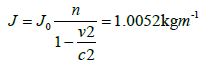(24)

= = 6.67408.10 11 ; 1.0049=6.707010-11; α2 0=6.69176·10-11

Now the error is greater than that between rest mass and electric charge, due to the fact that in the previous Equation (24) the gravitational constant is directly involved. This gravitational constant is the most difficult to be measured due to its small magnitude, despite being well known. The gravitational constant G has been previously calculated on the basis of Planck's constant h and the speed of light c . The permittivity 0, Planck's constant h and the fine structure constant have been previously calculated according to the speed of light c .

#### Conclusion

Rotation of Planck atoms in the fourth dimension gives rise to the electron charge; the energy of this rotation or electromagnetic field energy gives rise to the mass. The charge is the time it takes in returning in the fourth dimension.

Therefore, in the electron:

• Rotation in the fourth dimension, which originates the electric charge and so, the electric field is caused.

• Rotation in three-dimensional space generates the moving charge and therefore the magnetic field.

• The energy of the rotation, which we call mass, leads to the gravitational field.

It is evident that by changing the rotation direction, the polarity of the electric charge is changed. Clockwise rotation in the fourth dimension can only be cancelled with another anti-clockwise rotation, resulting from the Principle of Conservation of Charge.The mass of a Planck atom decreases with decreasing rotation, just as the electric charge increases and the wavelength or diameter (mprp=mλ). When increasing the wavelength, the travel speed decreases to the value α c. The fine structure constant relates the constant gravitational field G with constant magnetic field μ0.

G=α20

Gribbin was correct when he stated that the only alternative to the quantum probabilities, superposition of states, wave function collapse, and the spooky action at a distance, is that everything is predetermined .

#### References

Select your language of interest to view the total content in your interested language

### Article Usage

• Total views: 20510
• [From(publication date):
June-2016 - Feb 22, 2020]
• Breakdown by view type
• HTML page views : 20003Can't read the image? click here to refresh